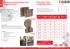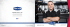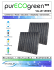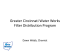# Determination of parameters for a half

## Transcription

Determination of parameters for a half
```Adam GRACZYK
Lodz University of Technology, Institute of Electrical Engineering Systems
doi:10.15199/48.2015.04.24
Determination of parameters for a half-band filter in a digital
Hilbert transformer in a reactive power measurement system
Abstract. This article presents a selection analysis of the parameters used for a half-band filter in a digital Hilbert transformer. The parameters were
determined for the transformer’s use in measuring Budeanu reactive power. This solution allowed to check the operation of the filter by comparing
the results obtained with the filter with the results of Budeanu reactive power obtained in the frequency domain. Fifty half-band filters were designed
and tested. As a result of the analysis the best filter was chosen. For this half-band filter the obtained values of the relative error of the reactive
power measurement were less than or equal to 0.01% for different types of load parameters, signals and sampling frequencies.
Streszczenie. W artykule przedstawiono analizę doboru parametrów filtra half-band, stosowanego w transformatorze Hilberta. Parametry te
określono dla transformatora zastosowanego do pomiaru mocy biernej według Budeanu. Rozwiązanie takie pozwoliło sprawdzić działanie filtra
porównując otrzymane z jego użyciem wyniki mocy z wynikami otrzymanymi w dziedzinie częstotliwości ze wzoru Budeanu. Do testów
zaprojektowano pięćdziesiąt filtrów Hilberta. Z nich wybrano filtr o najlepszych parametrach, dla których błąd pomiaru mocy biernej był mniejszy lub
równy 0.01% dla różnych sygnałów wejściowych, typów odbiornika i częstotliwości próbkowania. (Dobór parametrów filtra half-band dla
cyfrowego transformatora Hilberta w układzie do pomiaru mocy biernej).
Keywords: half-band filter, Hilbert transformer, reactive power measurement.
Słowa kluczowe: filtr half-band, transformator Hilberta, pomiar mocy biernej.
Introduction
The Hilbert transform converts an input real signal into a
different real signal, shifted by phase about -90 degrees for
0   < , and about 90 deg for -    < 0 in relation to the
input signal [1, 2]. All input signal frequency components
are shifted. The Hilbert transformer, which makes the actual
Hilbert transform, can operate in the frequency domain or in
the time domain. In the second case, the ideal Hilbert
transformer is a filter or a system of filters, whose frequency
response H(j) is given by
 j ,

H  j    0,
 j,

(1)
0
0
0
and the magnitude response by |H(j)| = 1 .
A Hilbert transformer can be used, amongst other
things, for measurement of reactive power in the time
domain according to the equation defined by Nowomiejski
[3, 4], which is described as follows:
(2)
Q
Q


i 1
i 1
U i I i sin i   Qi
where: Ui is the rms value of the voltage harmonic, Ii is the
rms value of the current harmonic, i is the phase angle
between the current and the voltage harmonics.
It can therefore be assumed that formula (2) expresses
Budeanu reactive power in the time domain.
parameters for a half-band filter used in a digital Hilbert
transformer. The parameters were determined for the
transformer’s use in measuring Budeanu reactive power in
the time domain, although at present reactive power is
determined from the definition of Iliovici [6, 7]. The aim here
104
Hilbert transformer
A Hilbert transformer can be realised using discrete
finite impulse response filters (FIR) or infinite impulse
response filters IIR [1, 2]. A different, interesting way to
realise the Hilbert transformer in domain time, is to use a
phase splitter [9, 10, 11]. This works as a system including
two all-pass filters F1 and F2 (Fig. 1), which phase
characteristics differ from each other by ca. -90 degrees.
The system does not create a signal directly equal to the
Hilbert transform of the input signal, but gives two output
signals, which are the mutual Hilbert transforms of each
other.
1   kT
 1   kT
u t H it dt 

 it H u t dt
kT 
kT 
where: H[u(t)], H[i(t)] – the voltage and current Hilbert
transforms.
Equation (2) gives the same results as for Budeanu
reactive power [4, 5] described in the formula
(3)
is the later development of a measurement system with a
phase shifter, with additional correction to convert the
results of Budeanu reactive power obtained in the time
domain into results obtainable by performing measurements
according to Iliovici’s definition. Work on such a system is
underway by the author and other researchers .
Fig. 1. Phase splitter.
The amplitude frequency characteristics of the filters F1
and F2 should satisfy |H1(ej)| = |H2(ej)| = 1 in the frequency
range (0, -0), where H1(ej) and H2(ej) are the transfer
functions of F1 and F2. The transfer functions should satisfy
the equation for the Hilbert phase-shift characteristic :
(4)
    j,
H e  
H1 e j
2
j
0       0
The all-pass filters can be realised as a cascade of the
low-order all-pass sections. A design method for IIR Hilbert
all-pass filters based on half-band filtering was presented by
Rashid [12, 13]. In this method, the half-band filter is
obtained by modifying the conventional elliptic filters, so that
PRZEGLĄD ELEKTROTECHNICZNY, ISSN 0033-2097, R. 91 NR 4/2015
all the poles of the half-band filter lie on an imaginary axis
. The transfer function of the half-band IIR filter  can
then be written as
(5)
 
 
H HB z   z 1H F 0 z 2  H F 2 z 2
where HF0(z2) and HF2(z2) are the all-pass filters.
The half-band filter has the frequency range (-p, p)
, where p < /2 is the circular passband edge
frequency. For the purpose of obtaining the band frequency
0 <  <  - 0, (0 > 0), it is necessary to perform a
frequency shift by /2. The shift is achieved by substituting z
for –jz into (5), which yields z-1HF0(-z2) and HF2(-z2) [11, 13].
The last transfer will constitute a pair of 90 degree phase
shifters. After the mathematical transformations, we obtain
the transfer functions of two digital all-pass filters HF1 (6)
and HF2 (7) , which can then be implemented in digital
signal processing (DSP) systems.
(6)
(7)
1
 z 2
a
H F1 z   z 1  i
1 2
ai 1 1 
z
ai
H F 2 z   
ai  z
2
ai 1 1  ai z
2
The procedure for calculating the coefficients ai is
described in literature [12, 15]. The computer algorithm
used to calculate the filter coefficients is presented in .
To make the calculations, the following parameters of the
half-band filter have to be brought in to the algorithm: the
passband edge (p) in units of , and the ripples of the
passband and the stop-band (p and s) [14, 15]. The actual
cut-off frequency 0 of the designed Hilbert transform may
be different than the frequency /2 - p. But it does satisfy
the formula 0  /2 - p , and depends on the half-band
filter parameters used. The usefulness of the half-band filter
in reactive power measurement also depends on its
parameters. The results obtained from simulations show
that small changes of the filter’s parameters’ values can
cause very large errors in reactive power measurement.
Therefore, it is essential to select the proper filter
parameters. In order to choose the best set of coefficients,
the Author performed a comparative analysis of 50 halfband filters with different parameters’ values, which are
described in the next point.
Model of the measurement system
A model of the reactive power measurement system
Blockset Toolboxes (Fig. 2). It consisted of a deformed
voltage source, an RLC load, blocks to measure the
instantaneous current and voltage values, analog-to-digital
converters and systems for recording signals.
Calculation of the reactive power using a fast Fourier
transform (FFT) and the Hilbert transformer were made in
Matlab. Suitable DSP algorithms were implemented in Mfiles. The code for the calculation of the reactive power
using equation (3), on the basis of 50 voltage and current
harmonics, and the formula
(8)
filtering of the load voltage signal through the all-pass filter
HF1 (6).
Q
1
N
Fig. 2. Diagram of the model of the measurement system.
The coefficients for the filters HF1 and HF2 were
calculated for the half-band filter parameters, given in Table
1. Six sets of the filters were designed for each specified
circular frequency p. Each set of half-band filters was
designed with the same values for p and s, which are
equal to 1e-2, 1e-3, 1e-4, 1e-5, 1e-6 and 1e-7 respectively
(for simplicity's sake, in the following text p = s = d).
Table 1. Half-band filter parameters used to
coefficients.
Cut-off
Designation of Low cut-off
frequency f0
frequency
the filters
of the halfcharacteristics of the Hilbert
band filter fp
given in
transformer
= fs/4 – f0
passband
Figures 3-11
where fs –
(related to
the
the circular
frequency
sampling
frequency
0) [Hz]
[Hz]
1
2
3
4
5
6
7
8
5
10
20
30
40
50
60
100
3195
3190
3180
3170
3160
3150
3140
3100
calculate their
Passband
edge circular
frequency p
of the halfband filter
[]
0.49922
0.49844
0.49688
0.49531
0.49375
0.49219
0.49063
0.48438
Additionally, two half-band filters were designed for d
equal to 1e-5 and f0 equal to 5.2 Hz and 5.5 Hz, the
necessity of which arose during the simulation. In total, 50
half-band filters were designed, but because of the limited
half-band filter, which gives the most accurate values of the
reactive power (chosen during the simulation tests) are
presented. Low cut-off frequencies f0 greater than 50 Hz
were also used in the model, which was designed to
examine how shifting of the frequency band of the Hilbert
transformer influences real processing characteristics and
measurement results. In practice, it is possible that the real
frequency bands of the half-band filters may be shifted due
to low accuracy of the electronic elements, or the limited
capabilities of the equipment used. Two voltage signals
generated in the model were used for testing, with the first
used to examine all of the designed Hilbert transforms. It
consisted of five odd harmonics and is described by
9
u s1 t   U k sin 2f k t 
N 1
(9)
n 0
where: k = 1, 3… 9, f1 = 50 Hz, U1 = 325.27 V, U3 = 32.527
V, U5 = 16.2635 V, U7 = 6.5054 V, U9 = 3.2557 V
The second signal consisted of eleven odd harmonics
and is described by
 iF2 n u F1 n 
where: iF2 is the discrete current signal received by filtering
of the load current signal by means of the all-pass filter HF2
(7), and uF1 is the discreet voltage signal received by
k 1
PRZEGLĄD ELEKTROTECHNICZNY, ISSN 0033-2097, R. 91 NR 4/2015
105
(10)
u s 2 t  
21
 U k sin 2f k t 
k 1
where: k = 1, 3... 21, f1 = 50 Hz, U1 = 325.27 V, U3 = 65.054
V, U5 = 48.7905 V, U7,9,11,13,15,17,19,21 = 32.527 V
This signal was used to test five filters chosen during the
first selection. Measurements were made for resistive (R),
resistive-inductive (RL) and resistive-capacitive (RC) loads.
In all cases, the passive elements were connected in series.
Tests with signal us1 were run for five sets of load
parameters. Tests with signal us2 were run for eight
resistive-inductive and six resistive-capacitive loads. All
load parameters are given in Table 2.
Table 2. The load parameters used for signals us1 and us2.
No. Set for us1
Set for us1
Set for us2
Set for us2
1
2
3
4
5
6
7
8
R [] L [mH] R []
10
10
10
100
10
10
0.1
C [mF] R []
2
10
60
10
10
10
10
10
10
10
L [mH]
0.1
0.5
1
5
10
50
100
500
R []
10
10
10
10
10
10
C [mF]
0.01
0.6
1
6
10
60
the model, calculations of the reactive power were also
made in the frequency domain on the basis of 50 voltage
and current harmonics, in every input signal period. This
required processing of the signals in a frequency band from
0 Hz to 2.5 kHz. The minimum sampling frequency that
satisfies the sampling theorem and enables collection of 2n
samples in every signal period is equal to 6.4 kHz. This
gives 128-point FFT at a resolution of 50 Hz. However, at
this sampling frequency the transition bandwidth of antialiasing filters is only equal to 0.7 kHz. A such, the sampling
frequency was increased to 12.8 kHz. This gave a 256-point
FFT and transition bandwidth of 3.9 kHz. This solution
allows for use of a lower order anti-aliasing filter. 18-bit
analogue-to-digital converters were used to acquire the
signals in the model.
Results
The results of the simulation tests obtained with signal
us1 are presented in Tables 3 and 4 and in Figures 3-10.
Due to the limited page count of this article, only a selection
of results are given. Figures 3-10 show the frequency
characteristics of the half-band filters for the different values
of their parameters. Figure 3 shows the amplitude
characteristics of the all-pass filters HF1 and HF2
respectively, for frequencies from 0 Hz to fs/2 Hz. This
relates to the filters designed for d = 1e-2. These have the
identical amplitude characteristics for all the cut-off
frequencies f0 of the Hilbert transformer (Table 1), which
were used to calculate the half-band filters.
The characteristics overlap and create one line in these
figures. It should be noted that neither of the all-pass filters
designed for the other d parameter values had signal
amplitude attenuation across the band from 0 Hz to fs/2 Hz.
The following figures present the phase characteristics
of the relationship between the transfer functions of the allpass filters HF1/HF2. This relationship characterises the
phase shift between the output signals of the two all-pass
filters. Its characteristics should be considered as the phase
characteristics of the Hilbert transformer. Figure 4 presents
the phase characteristics HF1/HF2 for d = 1e-2, for the
frequency range from 0 Hz to fs/2 Hz. As can be seen, the
phase characteristics are sloped, and deflect from -90
degrees at the beginning and end of the frequency band.
106
Fig. 3. Magnitude responses of the all-pass filters HF1 and HF2 for d
= 1e-2 for frequency range from 0 Hz to fs/2 Hz.
Fig. 4. Phase responses of the half-pass filter HF1/HF2 for d = 1e-2
for frequency range from 0 Hz to fs/2 Hz.
Fig. 5. Phase responses of the half-pass filter HF1/HF2 for d = 1e-2
for frequency range from 40 Hz to 100 Hz. Where 1 is the
characteristic for f0 = 5 Hz, 2 for f0 = 10 Hz, 3 for f0 = 20 Hz, 4 for f0
= 30 Hz, 6 for f0 = 50 Hz, and 7 for f0 = 60 Hz. Characteristics for f0
= 40 Hz (no. 5), and f0 = 100 Hz (no. 8) are out of the scale.
Furthermore, as is shown enlarged in Figure 5 the
characteristics have ripples, which introduce a deviation
from -90 degrees. The values given in Tables 3 and 4 show
that even the smallest deviations in the characteristics of
the half-pass filter can cause errors of even several percent
in the reactive power measurement. As such, it is important
to obtain not only the proper passband of the Hilbert
transformer, but also the small ripples in this band, too.
Figure 5 shows that in some cases the characteristics of
the half-band filter designed for the small low cut-off
frequency f0 of the Hilbert transformer passband do not
pass that close to -90 degrees at 50 Hz frequency. This
also applies to filters designed with the use of very small
values for ripples d, for which, however, this meant a
reduction in the deviation of phase characteristics.
PRZEGLĄD ELEKTROTECHNICZNY, ISSN 0033-2097, R. 91 NR 4/2015
This can be observed in Figures 6-10, which present the
phase characteristics of the half-pass filters HF1/HF2 with its
parameters’ different values.
reducing the ripples. This problem is because the reduction
of d increases the nonlinearity of the phase characteristics
of the half-band filters. For example, for f0 = 10 Hz and d =
1e-5, 1e-6, 1e-7 (Table 3), the values of the error of the
reactive power measurement were amounted to -25%, 21%
and -18% in relation to the results obtained from Equation
(3).
Fig. 6. Phase responses of the half-pass filter HF1/HF2 for d = 1e-3
for frequency range from 0 Hz to 500 Hz, Where 4 is the
characteristic for 30 Hz, 6 for f0 = 50 Hz, 7 for f0 = 60 Hz, and 8 for
f0 = 100 Hz.
Fig. 9. Phase responses of the half-pass filter HF1/HF2 for d = 1e-6
for frequency range from 10 Hz to 100 Hz. Where 1 is the
characteristic for f0 = 5 Hz, 2 for f0 = 10 Hz, 4 for f0 = 30 Hz, and 7
for f0 = 60 Hz.
Fig. 7. Phase responses of the half-pass filter HF1/HF2 for d = 1e-4
for frequency range from 10 Hz to 100 Hz. Where 1 is the
characteristic for f0 = 5 Hz, 2 for f0 = 10 Hz, 3 for f0 = 20 Hz, 4 for f0
= 30 Hz, and 6 for f0 = 50 Hz.
Fig. 10. Phase responses of the half-pass filter HF1/HF2 for d = 1e-7
for frequency range from 10 Hz to 100 Hz. Where 1 is the
characteristic for f0 = 5 Hz, 3 for f0 = 20 Hz, 4 for f0 = 30 Hz, 5 for f0
= 40 Hz and 6 for f0 = 50 Hz.
Table 3. The relative measurement error for R = 10  and C = 2
mF. The results were calculated relative to the value equal to 824.41 Var, obtained from Equation (3).
The relative measurement error [%]
f0 \ d
d =1e-2
1e-3
1e-4
1e-5
1e-6
1e-7
f0 = 5 Hz
18.97
-0.54
-15,22
0.00
10.44
0.00
10 Hz
-1.98
-0.31
-0,04
-24.51
20.79
-18.20
20 Hz
-6.86
0.07
0,06
-50.06
41.37
0.00
5.39 -116.31
0,07
74.26
0.00
0.00
0.89 -122,96
0.01
82.57
0.00
-125.9 -108.66
0.00
Fig. 8. Phase responses of the half-pass filter HF1/HF2 for d = 1e-5
for frequency range from 10 Hz to 100 Hz. Where 1 is the
characteristic for f0 = 5 Hz, 2 for f0 = 10 Hz, 5 for f0 = 40 Hz, and 7
for f0 = 60 Hz.
30 Hz
The maximum ripples of the phase characteristics
meeting the requirements for the width of the passband
were, respectively: ± 1.2 deg for d = 1e-2 (Fig. 5), ± 0.11
deg for d = 1e-3 (Fig. 6), ± 0.011 for d = 1e-4 (Fig. 7), ±
0.0012 for d = 1e-5 (Fig. 8), ± 0.00012 for d = 1e-6 (Fig. 9)
and ± 0.000015 for d = 1e-7 (Fig. 10).
The results of the measurement error for reactive power
calculated using the half-band filters for the various d and f0
(Tables 3 and 4) showed that a reduction in the value of
parameter d did not improve the results of the reactive
power measurement for each frequency f0, in spite of
100 Hz
40 Hz
217.01
50 Hz
12.20
199.41
-0,09
60 Hz
39.49
234.55
186,5
-0.47
-0.13 -111,43
-525.97 -421,56 -339.09 -277.44
232.00 -199,11
These results were worse than for d = 1e-2, 1e-3 and
1e-4. Still-worse results for the same frequency f0 are
shown in Table 4. The results obtained for the same filter
parameters were absolutely unacceptable. However, for
example, the use of d = 1e-7 and f0 = 20 Hz given in the two
above-quoted cases (Tables 3 and 4) returned
measurement error values equal to 0.00 %.
PRZEGLĄD ELEKTROTECHNICZNY, ISSN 0033-2097, R. 91 NR 4/2015
107
Table 4. The relative measurement error for R = 10  and L = 0.1
mH. The results were calculated relative to the value equal to
17.387 Var, obtained from Equation (3).
The relative measurement error [%]
f0 \ d
d =1e-2
1e-3
1e-4
1e-5
1e-6
1e-7
f0 = 5 Hz
-924
26,44
738
0,10
-508
-0,16
10 Hz
96.0
15,24
2,18
1187
-1013
882
20 Hz
333
-3,53
-2,88
2417
-2022
0.00
30 Hz
-262
5570
-3,39
-3645
-0,05
-0.01
40 Hz
-10872
-43.0
5884
-0,32
-4057
0.00
50 Hz
-593
-9963
4,60
6022
5209
-0,01
60 Hz
-1929
-11784
-9296
22,97
6,46
5340
100 Hz
23732
19369
15772 13014
109
9437
Similar comparisons can be found for the other
frequencies f0 and d. It should also be noted that in some
cases the values of the error were extremely great, which
eliminated the possibility of using certain sets of
parameters.
Comparative analysis of the phase characteristics of all
the Hilbert transformers made with the use of the half-band
filters and the obtained results (Figs. 3-10, Tables 3 and 4)
indicated that the smallest error values were obtained using
half-band filters with the following parameters: f0 = 5.2 Hz
and d = 1e-5 as well as f0 = 20Hz, 30 Hz, 40 Hz, 50 Hz and
d = 1e-7. It should be noted that when d = 1e-7 the filters for
f0 = 5 Hz and 10 Hz did not meet the established accuracy
criterion, although they did give the widest passband in the
transformer. However, the criterion was met by the halfband filter with a lower cut-off frequency f0 of 50 Hz, which
is the first harmonic. This confirmed the thesis that when
designing the Hilbert transformer using the half-band filter,
consideration should be given to the lowest frequency f0 as
this can lead to the large measurement errors, even at very
small d.
The five selected filters were also tested using signal
us2. All five enabled measurement of reactive power with the
maximum relative error ± 0.01% at all of the tested loads,
and for both test signals, us1 and us2, at the sampling
frequency of 12.8 kHz. In order to arrive at the most
accurate instrument, a maximum measurement error was
assumed equal to ± 0.01%.
The selected filters were also tested with other sampling
frequencies, equal to 10 kHz, 5 kHz, and 2.5 kHz. In all
cases, the obtained results for reactive power bore
comparison with the results obtained with the sampling
frequency equal to 12.8 kHz, and with the results obtained
from (3). Only four filters, for f0 = 20 Hz, 30 Hz, 40 Hz, 50
Hz and d = 1e-7, passed all tests. Of the four half-band
filters which met the accuracy criterion, the best filter – in
terms of the number of coefficients of all-pass filters – was
the half-band filter designed for the frequency f0 = 40 Hz
and d = 1e-7. The coefficients for this filter are given in
Table 5.
Table 5. Coefficients of the half-band filter with parameters f0 = 40
Hz and d = 1e-7.
iindex of the
coefficient ai
0
1
2
3
4
5
6
7
8
108
Values of the coefficients
of the all-pass filter HF1
12.77682203135281
3.71324473529900
2.05507244449140
1.49447541819616
1.25162489260591
1.13219541570260
1.06842199472485
1.03118313540206
1.00567856751073
Values of the coefficients
of the all-pass filter HF2
0.02037133289752
0.16506481726003
0.37957308924292
0.58419407116651
0.74051173143580
0.84593926035847
0.91268434039588
0.95455527596383
0.98269299296319
Conclusions
In this paper, a comparative analysis of Hilbert
transformers built using 50 half-band filters with different
parameters has been made. The author’s tests have
shown that small changes in the values of the filter
parameters have a significant influence on the accuracy of
signal shifts, and consequently on the accuracy of reactive
power measurement. Furthermore, in spite of reducing
ripples, decrease of the values of parameter d did not
always improve measurement results. The same is true of
frequency f0 – its reduction did not always cause an
increase in the accuracy of reactive power measurement.
Comparative analysis of the filters’ characteristics and the
reactive power measurement results helped to determine
the best half-band filter, for which the obtained values of the
relative error of the reactive power measurement were less
than or equal to 0.01% for all considered types of load
parameters, signals and sampling frequencies. This filter
was designed for the frequency f0 = 40 Hz and d = 1e-7. It
should be noted that it had better properties than the filters
designed for lower f0 frequencies.
REFERENCES
 Lyons, R., Understanding digital signal processing, Prentice
Hall, (2004).
 Zieliński, T. P., Digital signal processing. From theory to
practice, Wydawnictwa Komunikacji i Łączności, (2009).
 Nowomiejski, Z., Generalized theory of electrical power, Archiv
fur Elektrotechnik, 59, (1977), 183-189.
 Pasko, M., Power theory review for systems with distorted
signals, Proc. of EPN-2002, Poland, Zielona Góra, (2002), 1146.
 Budeanu, C.I., Puissance reactives et fictives, RGE, T.XXIII,
(1928), 762-773.
 Iliovici M., Definition et mesure de la puissance et de lenergie
reactives, Bull. Soc. Franc. Electr., 5, (1925), 931-954.
 IEEE Std 1459-2010: Standard Definitions for the
Measurement of Electric Power Quantities Under Sinusoidal,
Nonsinusoidal, Balanced, or Unbalanced Conditions, (2010).
 Papierz, K., Reactive power measurement using Hilbert
transformation in according with IEEE Std 1459-2010,
Informatyka, Automatyka, Pomiary w Gospodarce i Ochronie
Środowiska, 4, (2011) 15-18.
 Oppenheim, A.A., and Schafer, R.W., Digital signal processing,
Prentice-Hall, Inc., (1975).
 Pang, H., Wang, Z., and Chen, J., A measuring method of the
single-phase ac frequency, phase, and reactive power based
on the Hilbert filtering, IEEE Transactions on Instrumentation
and Measurement, 56, no. 3, (2007), 918-923.
 Johansson H., and Wanhammar L., Digital Hilbert transformers
composed of identical allpass subfilters, Proceedings of the
1998 IEEE International Symposium on Circuits and Systems,
USA, Monterey, CA, 5: (1998), 437–440.
 Ansari, R., Elliptic filter design for a class of generalized
halfband filters, IEEE Transactions on Acoustics, Speech and
Signal Processing, 33, (1985), no. 5, 1146-1150.
 Ansari, R., IIR discrete-time Hilbert transformers, IEEE
Transactions on Acoustics, Speech and Signal Processing, 35,
(1987), no. 8, 1116-1119.
 Jackson L., Digital filters and signal processing. Third edition
with Matlab exercises, Kluwer Academic Publishers, (1996).
 Pun, KP., da Franca, JE., and Azeredo-Leme, C., Circuit
design for wireless communications. Improved Techniques for
 Schussler, H.W., and Steffen, P., Halfband filters and Hilbert
transformers, Circuits Systems Signal Processing, 17, (1998),
no. 2, 137-164.
Author: dr inż. Adam Graczyk, Politechnika Łódzka, Wydział
Elektrotechniki, Elektroniki, Informatyki i Automatyki, Instytut
Systemów Inżynierii Elektrycznej, ul. B. Stefanowskiego 18/22, 90924 Łódź, e-mail: [email protected]
PRZEGLĄD ELEKTROTECHNICZNY, ISSN 0033-2097, R. 91 NR 4/2015
```

### PREPERATION AREAS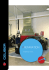### FleetValue ™ Oil Filters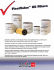### the latest offers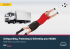### MADE IN THE USA - FreshWaterSystems.com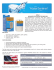### VENUS V-666 Full Face Mask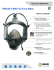### Airscreen 1000 14 x 24 Brochure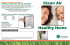### Combination Baffle/Mesh Filter Model Duo GFD GFD Baffle Filter